AP State Syllabus AP Board 9th Class Maths Solutions Chapter 3 The Elements of Geometry Ex 3.1 Textbook Questions and Answers.

## AP State Syllabus 9th Class Maths Solutions 3rd Lesson The Elements of Geometry Exercise 3.1

Question 1.
i) How many dimensions a solid has ?
Solution:
A solid has three dimensions namely length, breadth and height or depth.

ii) How many books are there in Euclid’s Elements ?
Solution:
There are 13 volumes in Euclid’s elements.iii) Write the number of faces of a cube and cuboid.
Solution:
Cube : 6 faces
Cuboid : 6 faces

iv) What is the sum of interior angles of a triangle ?
Solution:
The sum of interior angles of a triangle is 180°.

v) Write three undefined terms of geometry.
Solution:
Point, line and plane are three undefined terms in geometry.

Question 2.
State whether the following statements are true or false. Also give reasons for your answers.
a) Only one line can pass through a given point
b) All right angles are equal
c) Circles with same radii are equal
d) A finite line can be extended on its both sides endlessly to get a straight line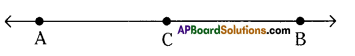e) From figure AB > AC
Solution:
a) Only one line can pass through a given point – False.
Reason : (Since, infinitely many lines can pass through a given point)
b) All right angles are equal – True.
c) Circles with same radii are equal – True.
d) A finite line can be extended on its both sides endlessly to get a straight line – True.
e) From figure AB > AC – True.Question 3.
In the figure given below, show that the length AH > AB + BC + CD.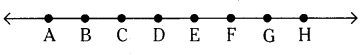Solution:
Given a line $$\stackrel{\leftrightarrow}{\mathrm{AH}}$$
To prove AH > AB + BC + CD
From the figure AB + BC + CD = AD
AD is a part of whole AH.
From Euclid’s axiom whole is greater than part.
⇒ AH > AB + BC + CD

Question 4.
If a point Q lies between two points P and R such PQ = QR, prove that PQ = $$\frac{1}{2}$$PR.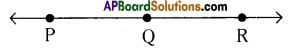Let PR be a given line.
Given that PQ = QR
i. e., Q is a point on PR.
⇒ PQ + QR = PR
⇒ PQ + PQ = PR [∵ PQ = QR]
⇒ 2PQ = PR
⇒ PQ = $$\frac{1}{2}$$ PR
Hence proved.Question 5.
Draw an equilateral triangle whose sides are 5.2 cm
Soluton:
Step – 1 : Draw a line segment AB of length 5.2 cm. *
Step – 2 : Draw an arc of radius 5.2 cm with centre A.
Step – 3 : Draw an arc of radius 5.2 cm with centre B.
Step – 4 : Two arcs intersect at C; join C to A and B.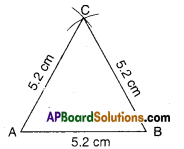Δ ABC is the required triangle.

Question 6.
What is a conjecture? Give an example for it.
Solution:
Mathematical statements which are neither proved nor disproved are called conjectures. Mathematical discoveries often start out as conjectures. This may be an educated guess based on observations.
Eg : Every even number greater than 4 can be written as sum of two primes. This example is called Gold Bach Conjecture

Question 7.
Mark two points P and Q. Draw a line through P and Q. Now how many lines are parallel to PQ, can you draw ?
Solution: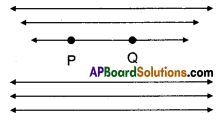Infinitely many lines parallel to PQ can be drawn.

Question 8.
In the figure given below, a line n falls on lines / and m such that the sum of the interior angles 1 and 2 is less than 180°, then what can you say about lines l and m ?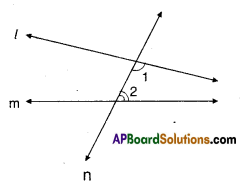Solution:
Given : l, m and n are lines, n is a transversal.
∠1 < 90°
∠2 < 90°
If the lines l and m are produced on the side where angles 1 and 2 are formed, they intersect at one point.Question 9.
In the figure given below, if ∠1 = ∠3, ∠2 = ∠4 and ∠3 = ∠4 write the rela-tion between ∠1 and ∠2 using Euclid’s postulate.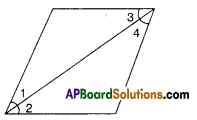Solution :
Given : ∠1 = ∠3
∠3 = ∠4
∠2 = ∠4
∴∠1 = ∠2

∵Both ∠1 and ∠2 are equal to ∠4. (By Euclid’s axiom things which are equal to same things are equal to one another).Question 10.
In the figure given below, we have BX = $$\frac{1}{2}$$ AB, BY= $$\frac{1}{2}$$ BC and AB = BC. Show that BX = BY.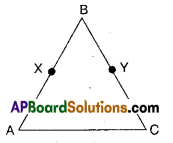Solution:
Given : BX = $$\frac{1}{2}$$ AB
BY = $$\frac{1}{2}$$BC
AB = BC
To prove : BX = BY
Proof: Given AB = BC [ ∵ By Euclid’s axiom things which are halves of the same things are equal to one another]
$$\frac{1}{2}$$ AB = $$\frac{1}{2}$$ BC
BX = BY
Hence proved.